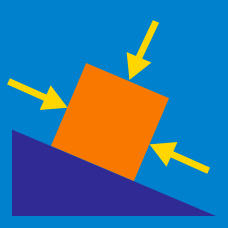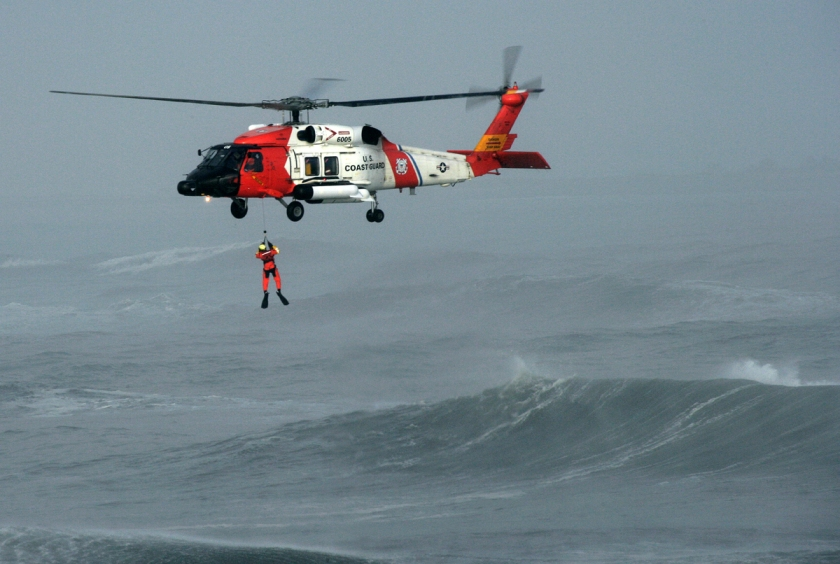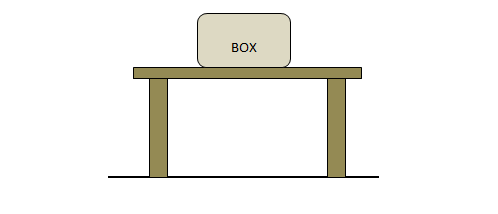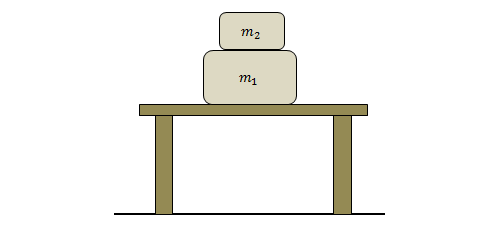Classical Mechanics

# Interactions between ObjectsA helicopter is rescuing a man by pulling him up on a rope. Which of the following best describes the forces that operate in this situation?

A man is swimming in water. If he swims at a constant speed, which of the following statements is/are true?

I. The man exerts a force on the water.

II. The water pushed by the man exerts a force on the man, generating thrust.

III. The water ahead of the man exerts a force on the man, generating resistance.In the figure above, there is a desk on the floor, and a box lies on the surface of the desk. If all of the objects in the figure are at rest, which of the following forces is different from the other three forces in magnitude?Two boxes of masses $$m_1=4\text{ kg},$$ and $$m_2=2\text{ kg}$$ are stacked on a tabletop, as shown in the figure above. Considering all the forces in this situation, which of the following is NOT the magnitude of one of these forces?

The gravitational acceleration is $$g=10\text{ m/s}^2.$$

A man stands still on the floor and feels two forces: the downward pull of gravity and the upward force from the floor.

Which of the following is correct about these two forces?

×

Problem Loading...

Note Loading...

Set Loading...